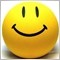iFractals in Mql5150

How to do this in Mql5

In mql4

val1 = iFractals(NULL, 0, MODE_UPPER, i);

val2 = iFractals(NULL, 0, MODE_LOWER, i);2100

scorpion007net:

How to do this in Mql5

In mql4

val1 = iFractals(NULL, 0, MODE_UPPER, i);

val2 = iFractals(NULL, 0, MODE_LOWER, i);

1. See mql5 iFractals

2. Declare some global variable

int    handle_fractal;              //--- for fractal handle
double fractal_up[],fractal_down[]; //--- for collecting fractal data

3. Initialize the handle and to make life easier set the array as timeseries.

handle_fractal = iFractals(_Symbol, PERIOD_CURRENT);

ArraySetAsSeries (fractal_up, true);
ArraySetAsSeries (fractal_down, true);

4. After checking if handle_fractal return INVALID_HANDLE, we can get the data using CopyBuffer, you can write this in OnTick() for EA, and OnCalculate for CI.

//--- check if there's error when creating iFractal
if (handle_fractal == INVALID_HANDLE)
{
handle_fractal = iFractals(_Symbol, PERIOD_CURRENT);
}
if (handle_fractal == INVALID_HANDLE) return;

//--- get the data
CopyBuffer(handle_fractal, UPPER_LINE, i, 1, fractal_up);
CopyBuffer(handle_fractal, LOWER_LINE, i, 1, fractal_down);

PS : I write the code using SRC Button, so it is not compiled or tested.150

Thanks..

It is Working!!

:)